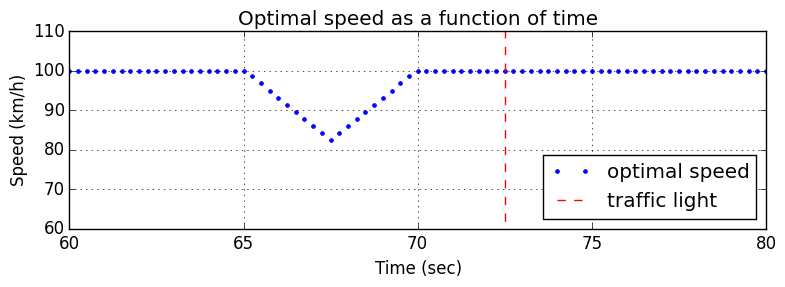## Riddler problem: can you time the stoplight just right?¶

This was posed as a Riddler problem on Jan. 20, 2017:

You are driving your car on a perfectly flat, straight road. You are the only one on the road and you can see anything ahead of you perfectly. At time t=0, you are at Point A, cruising along at a speed of 100 kilometers per hour, which is the speed limit for the whole road. You want to reach Point C, exactly 4 kilometers ahead, in the shortest time possible. But, at Point B, 2 kilometers ahead of you, there is a traffic light.

At time t=0, the light is green, but you don’t know how long it has been green. You do know that at the beginning of each second, there is a 1 percent chance that the light will turn yellow. Once it turns yellow, it remains yellow for 5 seconds and then turns red for 20 seconds. Your car can accelerate or decelerate at a maximum rate of 2 meters per second-squared. You must always drive at or below the speed limit. You can pass through the intersection when the traffic light is yellow, but not when it is red.

The code below uses integer programming to solve the problem under the approximation that yellow lights are relatively rare.

Laurent Lessard

In :
using JuMP, PyPlot, Gurobi

In :
Tmax = 160                 # max time of simulation (seconds)
dt = 0.25                   # discretization interval (seconds)

T = round(Int, Tmax/dt)    # total number of time steps
vmax = 100000/3600         # max speed, 100 km/h, converted to m/sec
amax = 2                   # max acceleration, 2 m/sec^2
xlight = 2000              # distance of the light, 2 km, converted to m.
xend = 4000                # distance of the finish, 4 km, converted to m.
ty = round(Int,5/dt)       # duration of the yellow light in timesteps
tr = round(Int,20/dt)      # duration of the red light in timesteps

m = Model(solver = GurobiSolver(OutputFlag=0))

# primary equations: assuming all goes right
@variable(m, 0 <= v[1:T] <= vmax)
@variable(m, -amax <= a[1:T] <= amax)
@variable(m, x[1:T] )

@constraint(m, x == 0)
@constraint(m, v == vmax)
@constraint(m, dveqn[t=1:T-1], v[t+1]-v[t] == a[t]*dt )
@constraint(m, dxeqn[t=1:T-1], x[t+1]-x[t] == v[t]*dt + 0.5*a[t]*dt^2 )

# contingency equations: assuming light turns yellow
@variable(m, 0 <= vhat[1:T,1:ty+tr] <= vmax)
@variable(m, -amax <= ahat[1:T,1:ty+tr] <= amax)
@variable(m, xhat[1:T,1:ty+tr])

@constraint(m, cxhat[t=1:T], xhat[t,1] == x[t])
@constraint(m, cvhat[t=1:T], vhat[t,1] == v[t])
@constraint(m, cdveqn[t=1:T,τ=1:ty+tr-1], vhat[t,τ+1]-vhat[t,τ] == ahat[t,τ]*dt )
@constraint(m, cdxeqn[t=1:T,τ=1:ty+tr-1], xhat[t,τ+1]-xhat[t,τ] == vhat[t,τ]*dt + 0.5*ahat[t,τ]*dt^2 )

# running a yellow light
@variable(m, zgo[t=1:T], Bin)
@constraint(m, xhat[:,ty] .>= xlight*zgo )

# stopping for a red light
@variable(m, zstop[t=1:T], Bin)
@constraint(m, xhat[:,ty+tr] .<= xlight + (1-zstop)*xend )

# logic constraint; one of them must happen!
@constraint(m, zgo + zstop .>= 1)

# termination criterion: are we there yet?
@variable(m, zfinish[1:T], Bin)
@constraint(m, x .>= xend*zfinish )

# objective function: reach the end as soon as possible assuming no ill-timed yellow lights.
@objective(m, Max, sum(zfinish) )
;

In :
@time solve(m)

 11.888435 seconds (279 allocations: 23.356 MB, 0.07% gc time)

Out:
:Optimal
In :
t_val = 0:dt:Tmax; t_val = t_val[1:end-1]
a_val = getvalue(a)
v_val = getvalue(v)*3.6
x_val = getvalue(x)/1000
z_fin = getvalue(zfinish)
z_go = getvalue(zgo)
z_stop = getvalue(zstop)
vhat_val = getvalue(vhat)*3.6
txlight = t_val[findmin(abs(x_val-xlight/1000))]
txend = t_val[findmin(abs(x_val-xend/1000))];

In :
figure(figsize=(8,3))
plot(t_val, v_val, ".")
plot(txlight*[1,1], [0,120], "r--")
plot(txend*[1,1], [0,120], "m--")
xlim([60,80]); ylim([60,110])
grid()
xlabel("Time (sec)"); ylabel("Speed (km/h)")
title("Optimal speed as a function of time")
legend(["optimal speed","traffic light"],loc="lower right")
tight_layout()
#savefig("traffic_light_plot.png")